## 8.5 Interpreting confidence intervals

Now that we’ve shown you how to construct confidence intervals using a sample drawn from a population, let’s now focus on how to interpret their effectiveness. The effectiveness of a confidence interval is judged by whether or not it contains the true value of the population parameter. Going back to our fishing analogy in Section 8.3, this is like asking, “Did our net capture the fish?”.

So, for example, does our percentile-based confidence interval of (1991.24, 1999.42) “capture” the true mean year $$\mu$$ of all US pennies? Alas, we’ll never know, because we don’t know what the true value of $$\mu$$ is. After all, we’re sampling to estimate it!

In order to interpret a confidence interval’s effectiveness, we need to know what the value of the population parameter is. That way we can say whether or not a confidence interval “captured” this value.

Let’s revisit our sampling bowl from Chapter 7. What proportion of the bowl’s 2400 balls are red? Let’s compute this:

bowl %>%
summarize(p_red = mean(color == "red"))
# A tibble: 1 x 1
p_red
<dbl>
1 0.375

In this case, we know what the value of the population parameter is: we know that the population proportion $$p$$ is 0.375. In other words, we know that 37.5% of the bowl’s balls are red.

As we stated in Subsection 7.3.3, the sampling bowl exercise doesn’t really reflect how sampling is done in real life, but rather was an idealized activity. In real life, we won’t know what the true value of the population parameter is, hence the need for estimation.

Let’s now construct confidence intervals for $$p$$ using our 33 groups of friends’ samples from the bowl in Chapter 7. We’ll then see if the confidence intervals “captured” the true value of $$p$$, which we know to be 37.5%. That is to say, “Did the net capture the fish?”.

### 8.5.1 Did the net capture the fish?

Recall that we had 33 groups of friends each take samples of size 50 from the bowl and then compute the sample proportion of red balls $$\widehat{p}$$. This resulted in 33 such estimates of $$p$$. Let’s focus on Ilyas and Yohan’s sample, which is saved in the bowl_sample_1 data frame in the moderndive package:

bowl_sample_1
# A tibble: 50 x 1
color
<chr>
1 white
2 white
3 red
4 red
5 white
6 white
7 red
8 white
9 white
10 white
# … with 40 more rows

They observed 21 red balls out of 50 and thus their sample proportion $$\widehat{p}$$ was 21/50 = 0.42 = 42%. Think of this as the “spear” from our fishing analogy.

Let’s now follow the infer package workflow from Subsection 8.4.2 to create a percentile-method-based 95% confidence interval for $$p$$ using Ilyas and Yohan’s sample. Think of this as the “net.”

#### 1. specify variables

First, we specify() the response variable of interest color:

bowl_sample_1 %>%
specify(response = color)
Error: A level of the response variable color needs to be specified for the success
argument in specify().

Whoops! We need to define which event is of interest! red or white balls? Since we are interested in the proportion red, let’s set success to be "red":

bowl_sample_1 %>%
specify(response = color, success = "red")
Response: color (factor)
# A tibble: 50 x 1
color
<fct>
1 white
2 white
3 red
4 red
5 white
6 white
7 red
8 white
9 white
10 white
# … with 40 more rows

#### 2. generate replicates

Second, we generate() 1000 replicates of bootstrap resampling with replacement from bowl_sample_1 by setting reps = 1000 and type = "bootstrap".

bowl_sample_1 %>%
specify(response = color, success = "red") %>%
generate(reps = 1000, type = "bootstrap")
Response: color (factor)
# A tibble: 50,000 x 2
# Groups:   replicate [1,000]
replicate color
<int> <fct>
1         1 white
2         1 white
3         1 white
4         1 white
5         1 red
6         1 white
7         1 white
8         1 white
9         1 white
10         1 red
# … with 49,990 more rows

Observe that the resulting data frame has 50,000 rows. This is because we performed resampling of 50 balls with replacement 1000 times and thus 50,000 = 50 $$\cdot$$ 1000. The variable replicate indicates which resample each row belongs to. So it has the value 1 50 times, the value 2 50 times, all the way through to the value 1000 50 times.

#### 3. calculate summary statistics

Third, we summarize each of the 1000 resamples of size 50 with the proportion of successes. In other words, the proportion of the balls that are "red". We can set the summary statistic to be calculated as the proportion by setting the stat argument to be "prop". Let’s save the result as sample_1_bootstrap:

sample_1_bootstrap <- bowl_sample_1 %>%
specify(response = color, success = "red") %>%
generate(reps = 1000, type = "bootstrap") %>%
calculate(stat = "prop")
sample_1_bootstrap
# A tibble: 1,000 x 2
replicate  stat
<int> <dbl>
1         1  0.32
2         2  0.42
3         3  0.44
4         4  0.4
5         5  0.44
6         6  0.52
7         7  0.38
8         8  0.44
9         9  0.34
10        10  0.42
# … with 990 more rows

Observe there are 1000 rows in this data frame and thus 1000 values of the variable stat. These 1000 values of stat represent our 1000 replicated values of the proportion, each based on a different resample.

#### 4. visualize the results

Fourth and lastly, let’s compute the resulting 95% confidence interval.

percentile_ci_1 <- sample_1_bootstrap %>%
get_confidence_interval(level = 0.95, type = "percentile")
percentile_ci_1
# A tibble: 1 x 2
2.5% 97.5%
<dbl>   <dbl>
1    0.3    0.56

Let’s visualize the bootstrap distribution along with the percentile_ci_1 percentile-based 95% confidence interval for $$p$$ in Figure 8.26. We’ll adjust the number of bins to better see the resulting shape. Furthermore, we’ll add a dashed vertical line at Ilyas and Yohan’s observed $$\widehat{p}$$ = 21/50 = 0.42 = 42% using geom_vline().

sample_1_bootstrap %>%
visualize(bins = 15) +
geom_vline(xintercept = 0.42, linetype = "dashed")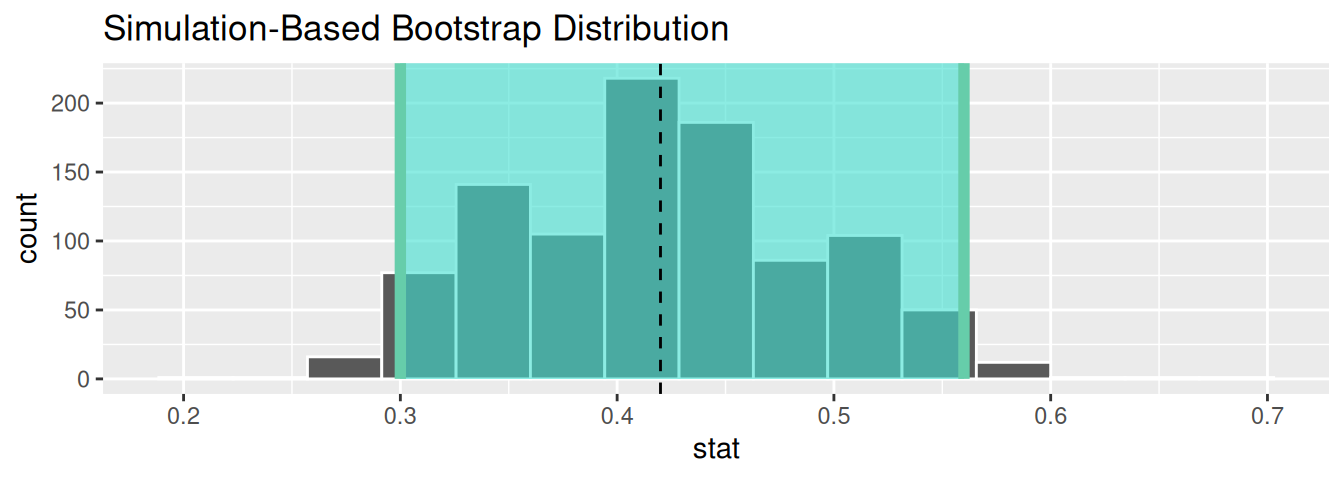FIGURE 8.26: Bootstrap distribution.

Did Ilyas and Yohan’s net capture the fish? Did their 95% confidence interval for $$p$$ based on their sample contain the true value of $$p$$ of 0.375? Yes! 0.375 is between the endpoints of their confidence interval (0.3, 0.56).

However, will every 95% confidence interval for $$p$$ capture this value? In other words, if we had a different sample of 50 balls and constructed a different confidence interval, would it necessarily contain $$p$$ = 0.375 as well? Let’s see!

Let’s first take a different sample from the bowl, this time using the computer as we did in Chapter 7:

bowl_sample_2 <- bowl %>% rep_sample_n(size = 50)
bowl_sample_2
# A tibble: 50 x 3
# Groups:   replicate 
replicate ball_ID color
<int>   <int> <chr>
1         1    1665 red
2         1    1312 red
3         1    2105 red
4         1     810 white
5         1     189 white
6         1    1429 white
7         1    2294 red
8         1    1233 white
9         1    1951 white
10         1    2061 white
# … with 40 more rows

Let’s reapply the same infer functions on bowl_sample_2 to generate a different 95% confidence interval for $$p$$. First, we create the new bootstrap distribution and save the results in sample_2_bootstrap:

sample_2_bootstrap <- bowl_sample_2 %>%
specify(response = color,
success = "red") %>%
generate(reps = 1000,
type = "bootstrap") %>%
calculate(stat = "prop")
sample_2_bootstrap
# A tibble: 1,000 x 2
replicate  stat
<int> <dbl>
1         1  0.48
2         2  0.38
3         3  0.32
4         4  0.32
5         5  0.34
6         6  0.26
7         7  0.3
8         8  0.36
9         9  0.44
10        10  0.36
# … with 990 more rows

We once again compute a percentile-based 95% confidence interval for $$p$$:

percentile_ci_2 <- sample_2_bootstrap %>%
get_confidence_interval(level = 0.95, type = "percentile")
percentile_ci_2
# A tibble: 1 x 2
2.5% 97.5%
<dbl>   <dbl>
1    0.2    0.48

Does this new net capture the fish? In other words, does the 95% confidence interval for $$p$$ based on the new sample contain the true value of $$p$$ of 0.375? Yes again! 0.375 is between the endpoints of our confidence interval (0.2, 0.48).

Let’s now repeat this process 100 more times: we take 100 virtual samples from the bowl and construct 100 95% confidence intervals. Let’s visualize the results in Figure 8.27 where:

1. We mark the true value of $$p = 0.375$$ with a vertical line.
2. We mark each of the 100 95% confidence intervals with horizontal lines. These are the “nets.”
3. The horizontal line is colored grey if the confidence interval “captures” the true value of $$p$$ marked with the vertical line. The horizontal line is colored black otherwise.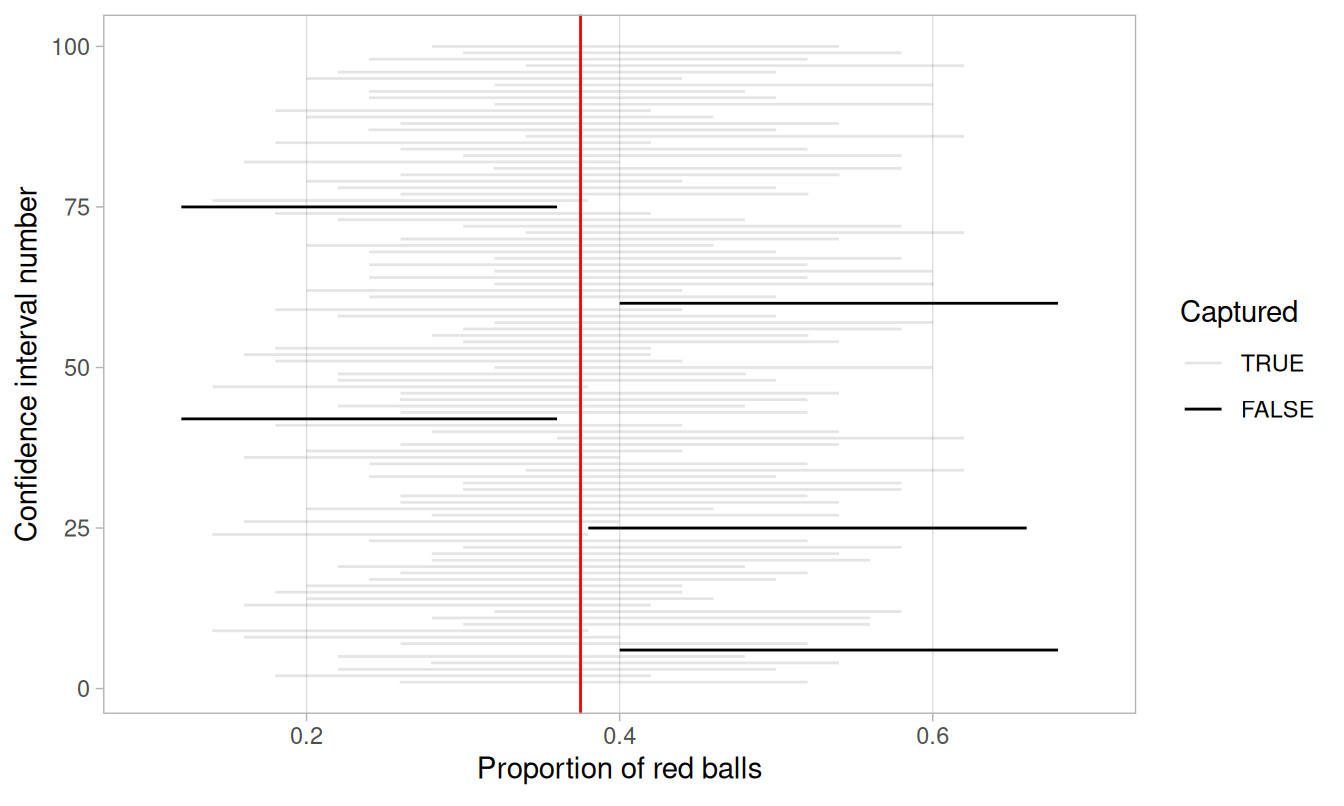FIGURE 8.27: 100 percentile-based 95% confidence intervals for $$p$$.

Of the 100 95% confidence intervals, 95 of them captured the true value $$p = 0.375$$, whereas 5 of them didn’t. In other words, 95 of our nets caught the fish, whereas 5 of our nets didn’t.

This is where the “95% confidence level” we defined in Section 8.3 comes into play: for every 100 95% confidence intervals, we expect that 95 of them will capture $$p$$ and that five of them won’t.

Note that “expect” is a probabilistic statement referring to a long-run average. In other words, for every 100 confidence intervals, we will observe about 95 confidence intervals that capture $$p$$, but not necessarily exactly 95. In Figure 8.27 for example, 95 of the confidence intervals capture $$p$$.

To further accentuate our point about confidence levels, let’s generate a figure similar to Figure 8.27, but this time constructing 80% standard-error method based confidence intervals instead. Let’s visualize the results in Figure 8.28 with the scale on the x-axis being the same as in Figure 8.27 to make comparison easy. Furthermore, since all standard-error method confidence intervals for $$p$$ are centered at their respective point estimates $$\widehat{p}$$, we mark this value on each line with dots.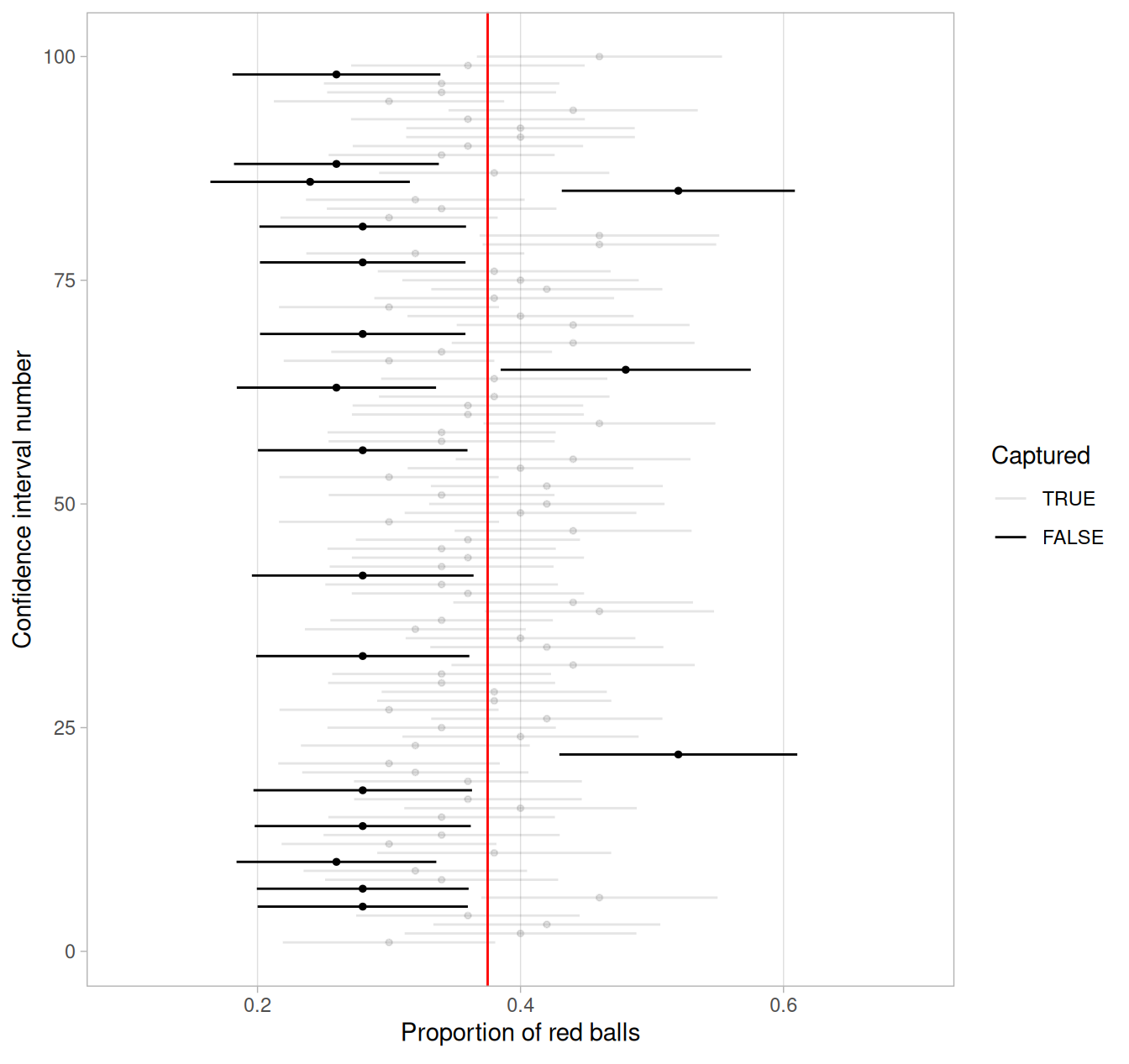FIGURE 8.28: 100 SE-based 80% confidence intervals for $$p$$ with point estimate center marked with dots.

Observe how the 80% confidence intervals are narrower than the 95% confidence intervals, reflecting our lower degree of confidence. Think of this as using a smaller “net.” We’ll explore other determinants of confidence interval width in the upcoming Subsection 8.5.3.

Furthermore, observe that of the 100 80% confidence intervals, 82 of them captured the population proportion $$p$$ = 0.375, whereas 18 of them did not. Since we lowered the confidence level from 95% to 80%, we now have a much larger number of confidence intervals that failed to “catch the fish.”

### 8.5.2 Precise and shorthand interpretation

Let’s return our attention to 95% confidence intervals. The precise and mathematically correct interpretation of a 95% confidence interval is a little long-winded:

Precise interpretation: If we repeated our sampling procedure a large number of times, we expect about 95% of the resulting confidence intervals to capture the value of the population parameter.

This is what we observed in Figure 8.27. Our confidence interval construction procedure is 95% reliable. That is to say, we can expect our confidence intervals to include the true population parameter about 95% of the time.

A common but incorrect interpretation is: “There is a 95% probability that the confidence interval contains $$p$$.” Looking at Figure 8.27, each of the confidence intervals either does or doesn’t contain $$p$$. In other words, the probability is either a 1 or a 0.

So if the 95% confidence level only relates to the reliability of the confidence interval construction procedure and not to a given confidence interval itself, what insight can be derived from a given confidence interval? For example, going back to the pennies example, we found that the percentile method 95% confidence interval for $$\mu$$ was (1991.24, 1999.42), whereas the standard error method 95% confidence interval was (1991.35, 1999.53). What can be said about these two intervals?

Loosely speaking, we can think of these intervals as our “best guess” of a plausible range of values for the mean year $$\mu$$ of all US pennies. For the rest of this book, we’ll use the following shorthand summary of the precise interpretation.

Short-hand interpretation: We are 95% “confident” that a 95% confidence interval captures the value of the population parameter.

We use quotation marks around “confident” to emphasize that while 95% relates to the reliability of our confidence interval construction procedure, ultimately a constructed confidence interval is our best guess of an interval that contains the population parameter. In other words, it’s our best net.

So returning to our pennies example and focusing on the percentile method, we are 95% “confident” that the true mean year of pennies in circulation in 2019 is somewhere between 1991.24 and 1999.42.

### 8.5.3 Width of confidence intervals

Now that we know how to interpret confidence intervals, let’s go over some factors that determine their width.

#### Impact of confidence level

One factor that determines confidence interval widths is the pre-specified confidence level. For example, in Figures 8.27 and 8.28, we compared the widths of 95% and 80% confidence intervals and observed that the 95% confidence intervals were wider. The quantification of the confidence level should match what many expect of the word “confident.” In order to be more confident in our best guess of a range of values, we need to widen the range of values.

To elaborate on this, imagine we want to guess the forecasted high temperature in Seoul, South Korea on August 15th. Given Seoul’s temperate climate with four distinct seasons, we could say somewhat confidently that the high temperature would be between 50°F - 95°F (10°C - 35°C). However, if we wanted a temperature range we were absolutely confident about, we would need to widen it.

We need this wider range to allow for the possibility of anomalous weather, like a freak cold spell or an extreme heat wave. So a range of temperatures we could be near certain about would be between 32°F - 110°F (0°C - 43°C). On the other hand, if we could tolerate being a little less confident, we could narrow this range to between 70°F - 85°F (21°C - 30°C).

Let’s revisit our sampling bowl from Chapter 7. Let’s compare $$10 \cdot 3 = 30$$ confidence intervals for $$p$$ based on three different confidence levels: 80%, 95%, and 99%.

Specifically, we’ll first take 30 different random samples of size $$n$$ = 50 balls from the bowl. Then we’ll construct 10 percentile-based confidence intervals using each of the three different confidence levels.

Finally, we’ll compare the widths of these intervals. We visualize the resulting confidence intervals in Figure 8.29 along with a vertical line marking the true value of $$p$$ = 0.375.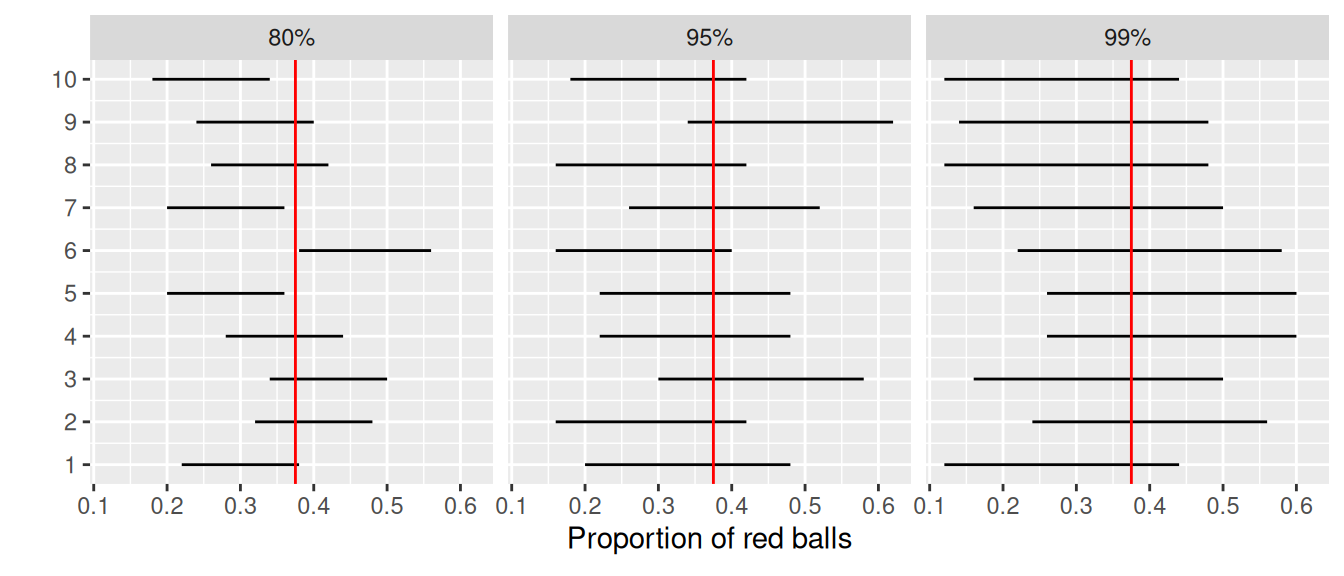FIGURE 8.29: Ten 80, 95, and 99% confidence intervals for $$p$$ based on $$n = 50$$.

Observe that as the confidence level increases from 80% to 95% to 99%, the confidence intervals tend to get wider as seen in Table 8.2 where we compare their average widths.

TABLE 8.2: Average width of 80, 95, and 99% confidence intervals
Confidence level Mean width
80% 0.162
95% 0.262
99% 0.338

So in order to have a higher confidence level, our confidence intervals must be wider. Ideally, we would have both a high confidence level and narrow confidence intervals. However, we cannot have it both ways. If we want to be more confident, we need to allow for wider intervals. Conversely, if we would like a narrow interval, we must tolerate a lower confidence level.

The moral of the story is: Higher confidence levels tend to produce wider confidence intervals. When looking at Figure 8.29 it is important to keep in mind that we kept the sample size fixed at $$n$$ = 50. Thus, all $$10 \cdot 3 = 30$$ random samples from the bowl had the same sample size. What happens if instead we took samples of different sizes? Recall that we did this in Subsection 7.2.4 using virtual shovels with 25, 50, and 100 slots.

#### Impact of sample size

This time, let’s fix the confidence level at 95%, but consider three different sample sizes for $$n$$: 25, 50, and 100. Specifically, we’ll first take 10 different random samples of size 25, 10 different random samples of size 50, and 10 different random samples of size 100. We’ll then construct 95% percentile-based confidence intervals for each sample. Finally, we’ll compare the widths of these intervals. We visualize the resulting 30 confidence intervals in Figure 8.30. Note also the vertical line marking the true value of $$p$$ = 0.375.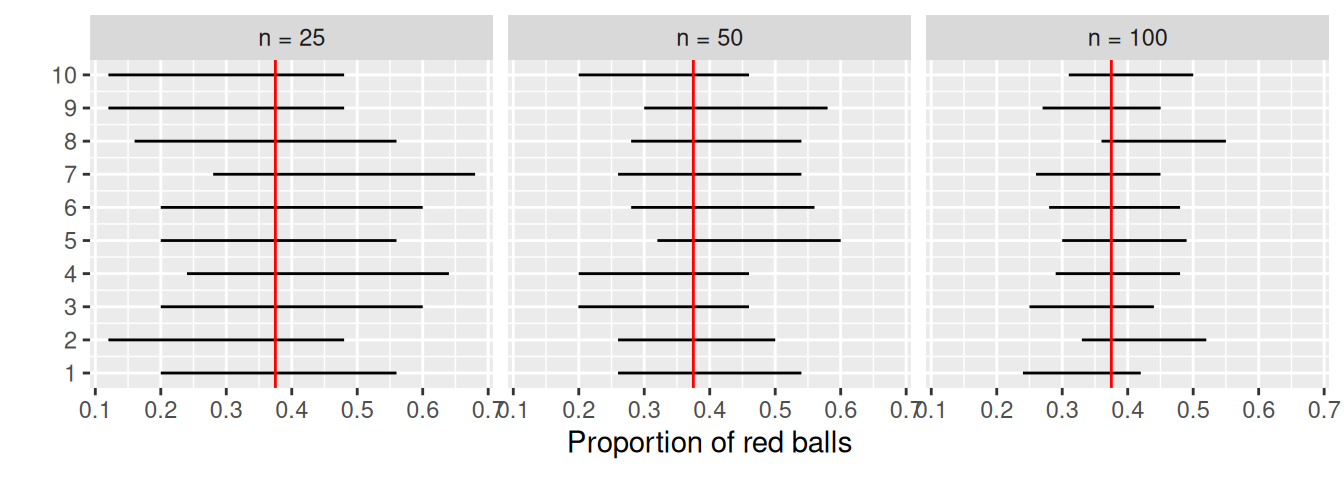FIGURE 8.30: Ten 95% confidence intervals for $$p$$ with $$n = 25, 50,$$ and $$100$$.

Observe that as the confidence intervals are constructed from larger and larger sample sizes, they tend to get narrower. Let’s compare the average widths in Table 8.3.

TABLE 8.3: Average width of 95% confidence intervals based on $$n = 25$$, $$50$$, and $$100$$
Sample size Mean width
n = 25 0.380
n = 50 0.268
n = 100 0.189

The moral of the story is: Larger sample sizes tend to produce narrower confidence intervals. Recall that this was a key message in Subsection 7.3.3. As we used larger and larger shovels for our samples, the sample proportions red $$\widehat{p}$$ tended to vary less. In other words, our estimates got more and more precise.

Recall that we visualized these results in Figure 7.15, where we compared the sampling distributions for $$\widehat{p}$$ based on samples of size $$n$$ equal 25, 50, and 100. We also quantified the sampling variation of these sampling distributions using their standard deviation, which has that special name: the standard error. So as the sample size increases, the standard error decreases.

In fact, the standard error is another related factor in determining confidence interval width. We’ll explore this fact in Subsection 8.7.2 when we discuss theory-based methods for constructing confidence intervals using mathematical formulas. Such methods are an alternative to the computer-based methods we’ve been using so far.